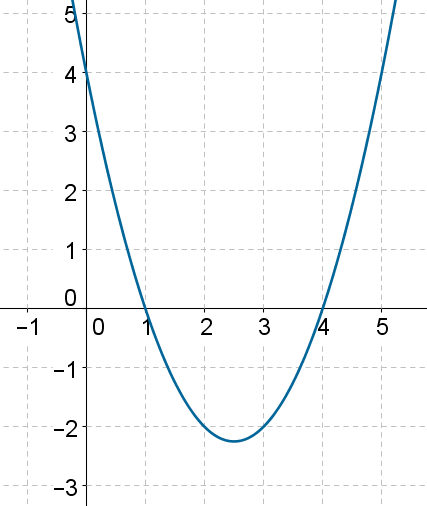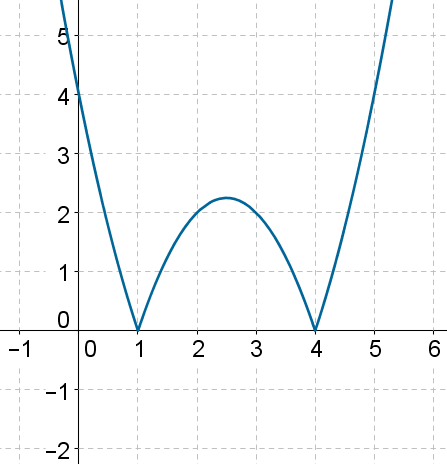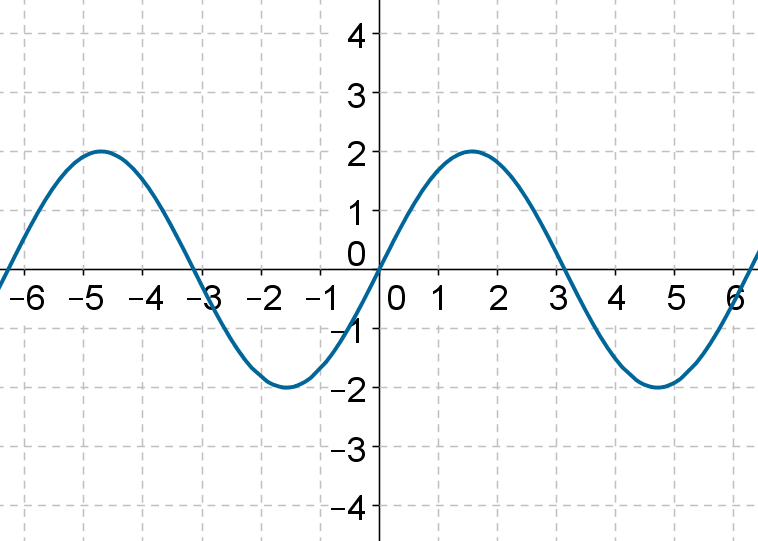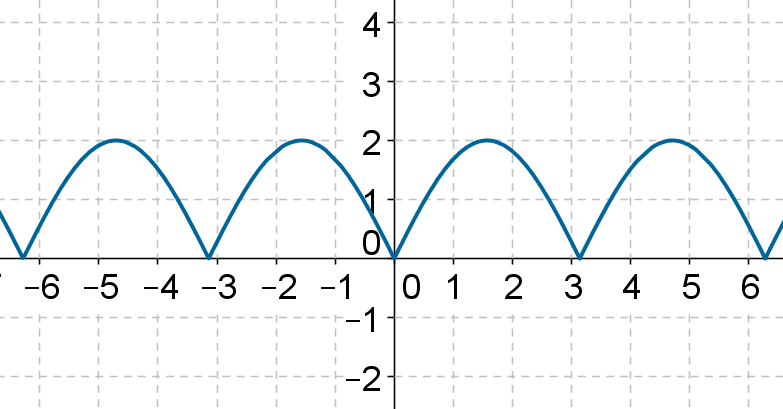# Applying modulus

Applying modulus

The following figure shows the graph of the function $$f\left( x \right) = {x^2} - 5x+ 4$$:Using this graph, can we plot the graph of $$y = g\left( x \right) = \left|{f\left( x \right)} \right|$$? The answer is simple: wherever f is positive, we leave the curve untouched; wherever f is negative, we reflect that part in the x-axis, because the effect of the modulus operation is to give us the positive magnitude. In this case, the graph of $$y = \left| {f\left( x \right)} \right|$$ will be as follows:Thus, the part of the curve below the x-axis in the original graph gets reflect in the x-axis.

Let’s see another example of this transformation. The following is the graph of $$y= f\left( x \right)$$, where f is some arbitrary function:And, the following is the graph of $$y = \left| {f\left( x \right)} \right|$$:Functions
Functions
grade 10 | Questions Set 2
Functions
Functions
grade 10 | Questions Set 1
More Important Topics
Numbers
Algebra
Geometry
Measurement
Money
Data
Trigonometry
Calculus
More Important Topics
Numbers
Algebra
Geometry
Measurement
Money
Data
Trigonometry
Calculus
Learn from the best math teachers and top your exams

• Live one on one classroom and doubt clearing
• Practice worksheets in and after class for conceptual clarity
• Personalized curriculum to keep up with school